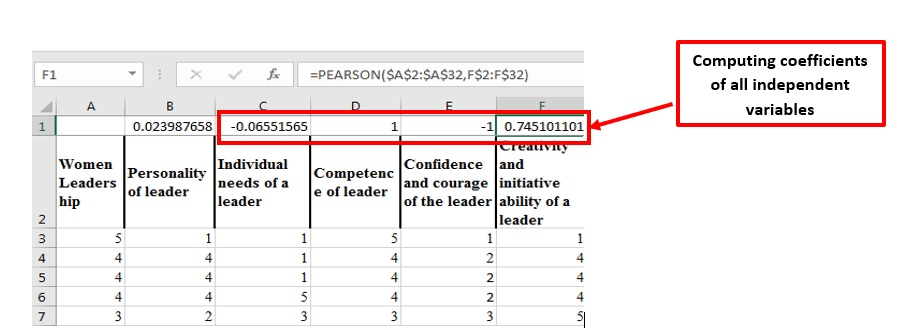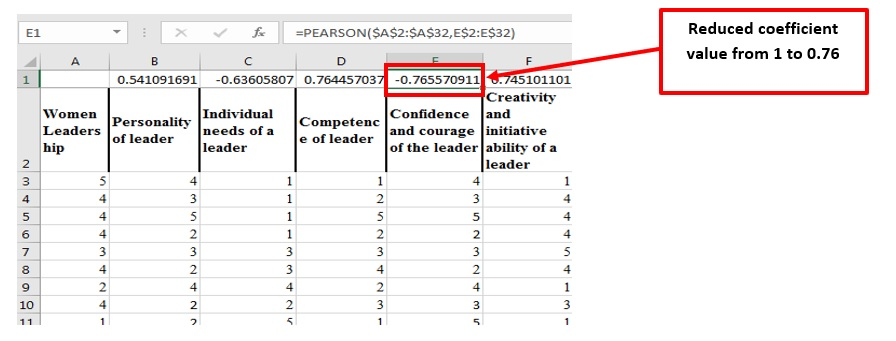# How to improve the correlation between the variables?

By Riya Jain & Priya Chetty on February 27, 2020
Photo by energepic.com from Pexels

Correlation refers to the extent of a relationship between the variables. In order to determine this relationship, it is essential to establish a correlation between the variables. For example, a researcher intends to find out how personal factors affect the success of women leaders. For this, the researcher needs to identify the determinants (variables) of personal factors like:

• needs,
• marital status,
• age,
• confidence,
• skills,
• innovativeness, etc.

This will then need to be tested individually or collectively against their success.

A previous article showed the process of conducting a correlation test. It also showed the condition required for proving a significant linkage between the dependent and independent variables. However, the dataset is not always perfect. When there are many outliers in the dataset or inconsistencies, a correlation cannot be determined. This is when the dataset needs processing.

This article shows how to process a dataset so that these inconsistencies can be removed, and correlation is proven between variables.

## What influences the significance (p) value?

The significance (p) value of Pearson’s correlation test value reveals that at least there is the existence of a moderate linear relationship between the variables. The coefficient value of correlation is dependent on the difference between the observations of variables, thus the essential requirement for the derivation of a high correlation value is:

• To maximize the difference between the variables or factors (or dependent and independent variables) in case of deriving a negative relationship
• To minimize the difference between the factors or variables (or dependent and independent variables) for obtaining a positive relationship

## Why use data processing?

Many a-times the value of correlation derived from the primary data is ‘perfect’, i.e 1 or -1. A Pearson correlation coefficient value of 1 or -1 signifies that there is an existence of a perfect positive or negative relationship between two variables. In the primary research, data is based on human perception of issues. Due to the presence of randomness and biases in human behaviour, a perfect correlation between the two variables is practically impossible. Some of the reasons for this bias include:

• Acquiescence or Friendliness bias: giving response just for completing the survey,
• Social desirability or Acceptability bias: answers to sensitive or personal questions based on social desirability,
• Habituation bias: the same answer to similarly worded questions, or
• Sponsor bias: influence of answer from the reputation or mission of the researcher.

Hence, there is a need to reduce or increase the correlation value. This is done through data processing.

## What is the procedure of data processing?

In order to process the dataset, it is initially recommended to check the Pearson correlation coefficient value in MS Excel before processing the dataset with any other software for analysis.

### Step 1: Determining the correlation value

Apply the Pearson correlation coefficient formulae and determine the value of linkage between the variables:

`Formula = PEARSON(Array 1, Array 2)`

## OR

`Formula = PEARSON(Dependent variable array, Independent variables array)`

For example: following the above-stated example, the Pearson value for the women leadership (dependent variable) and other independent variables is computed as shown below.Figure 1: Pearson Coefficient Value computation in MS Excel

### Step 2: Changing values of multiple independent variables

Note: In the case of many independent variables fix the dependent variable array and change the independent variable column by using \$ as shown below.

Now, drag the cursor from the extreme right corner towards other independent variables. The Pearson coefficient value for each linkage is shown in the below figure.Figure 3: Computed correlation coefficient values of all independent variables

As the above figure shows that the value of 1st (Personality of a leader) is 0.029, 2nd (individual needs of a leader) is approximately -0.066, and 4th variable (confidence and courage of leader) is -1. In contrast, the value for the 3rd variable (competence of leader) is too high i.e. 1. This data processing needs to be done for these 4 variables while the 5th variable (creativity and initiative ability of a leader) linkage is appropriate.

### Step 3: Processing the data

#### Case 1 (a): When the coefficient value is less than the moderate positive relationship value i.e. r < |0.5|

Figure 3 above shows a very large difference between the values of dependent and independent variables, like 5 and 1, or 4 and 1. In order to reduce this difference replace some of the observation values of the independent variable with the value close to the dependent variable’s.

When these values are changed, the coefficient automatically changes. This process is repeated until at least a 0.5 value of the Pearson coefficient is derived.Figure 4: Modified correlation coefficient results showing a positive relationship

This process is repeated for other independent variables that have a correlation value less than |0.5|.

#### Case 1 (b): When the coefficient value is less than the moderate negative relationship value i.e. r < |0.5|

On the other hand, when the correlation coefficient value is negative, i.e. less than 0 then two variables are said to be negatively correlated. To improve this correlation, increase the difference between the variables. This is done by identifying the independent variable observation, which is identical or close to the dependent observation value, and replacing it with the value which would increase the difference between the variables.

Follow this process for all variables showing a coefficient of less than -0.05.

### Case 2 (a): When the coefficient value is perfectly positive i.e. r = |1|

Figure 5 above shows that there is a perfect linkage between the variables i.e. women’s leadership and competence of leaders i.e. the values of dependent and independent variables are the same. The coefficient value is 1. It implies that the data is biased. In order to remove the bias, reduce the coefficient value by increasing the difference between the two variables’ observations.

This process is repeated until the correlation value is reduced to 0.8 or 0.9.Figure 2: Modified correlation coefficient results in a perfectly positive correlation.

## Case 2 (b): When the coefficient value is perfectly negative i.e. r = |-1|

The other way of reducing the correlation between the variables is by reducing the difference between the variables. In this case, the value of the independent variable is replaced by the value close to the dependent variable value.Figure 7: When the coefficient value is perfectly negative i.e. r = |-1|

The above-stated process is repeated until adequate linkage between the variables is derived.

## Performing the correlation analysis in SPSS

The final step in determining the linkage between the dependent and independent variables is to statistically analyze the dataset. The procedure used for Pearson correlation analysis in SPSS is followed and finally, a significant conclusion could be drawn by interpreting the results derived from the analysis.

Table 1: Correlation Analysis results

The above analysis thus shows that as the significance value of all the variables is less than the significance level of the study thus, there exists a linear relationship between women’s leadership and the confidence and courage of the leader.